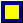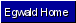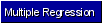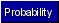Egwald Statistics: Probability and Stochastic Processes by Elmer G. Wiens Egwald's popular web pages are provided without cost to users. Please show your support by joining Egwald Web Services as a Facebook Fan:Follow Elmer Wiens on Twitter:Probability Distributions. A. Normal Distribution: N(µ, ø^2) Let µ = mean; ø2 = variance; ø = standard deviation. Then the density of the normal distribution is: f(x) = exp[-0.5 * ((x - µ)/ ø )^2)] / (2* pi * ø2)^.5 Density of the Normal Distribution with µ = 0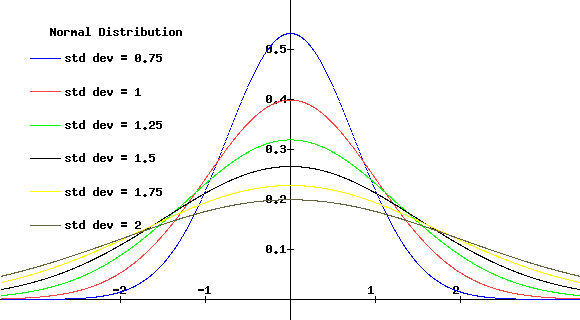When µ = 0 and ø^2 = 1, the distribution is called the standard normal distribution, denoted by N(0, 1) If the random variable X has a N(µ,ø^2) distribution, then the random variable Z = (X - µ)/ø has a N(0,1) distribution. B. Gamma Distribution Let the parameters of the gamma distribution be alfa and beta. Then the gamma density is: f(x) = K * alfa^beta * x^(beta-1) * exp(-alfa * x) where K = 1 / G(beta) and G = the gamma function. Density of the Gamma Distribution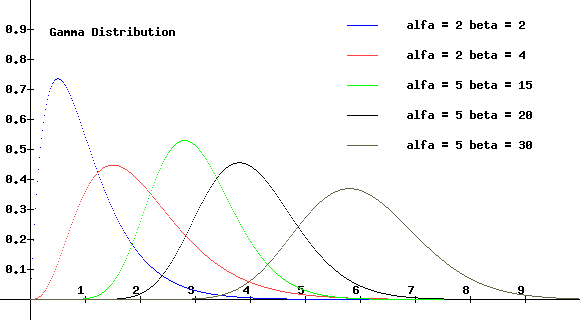C. Gamma Function The gamma function generally involves an integral. But, if n is an integer then: G(n) = (n - 1)! G(n + 1/2) = sqrt(pi) * (2*n)! / [(4^n) * n!)]Copyright © Elmer G. Wiens:   Egwald Web ServicesAll Rights Reserved.    Inquiries